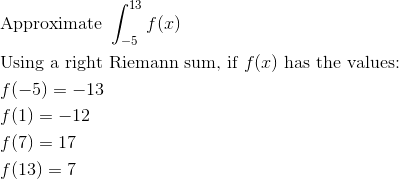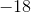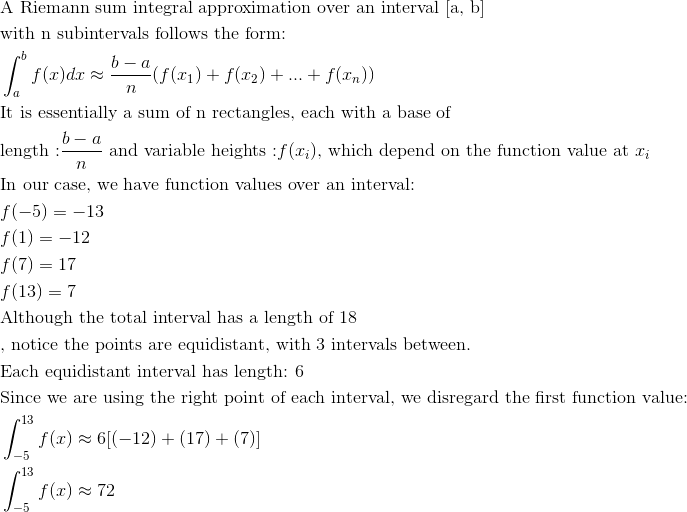AP Calculus AB : Riemann sums (left, right, and midpoint evaluation points)

Example Questions

← Previous 1 3

Example Question #1 : Riemann Sums (Left, Right, And Midpoint Evaluation Points)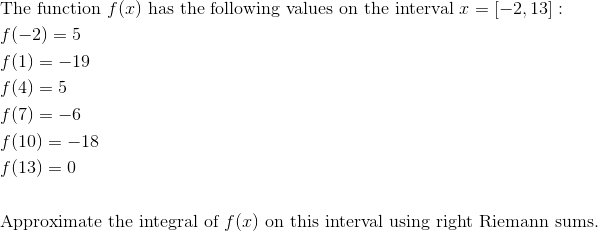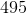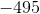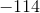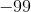Explanation: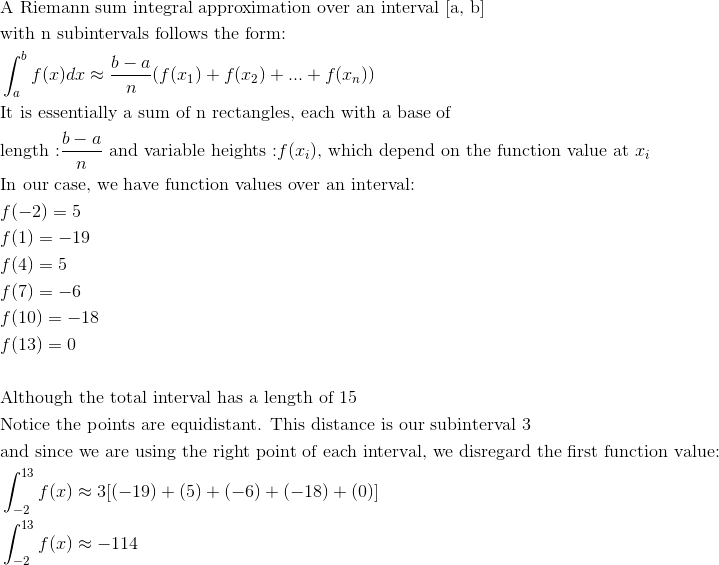Example Question #2 : Riemann Sums (Left, Right, And Midpoint Evaluation Points)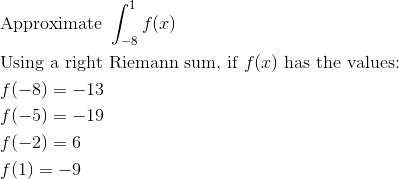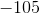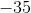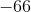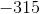Explanation: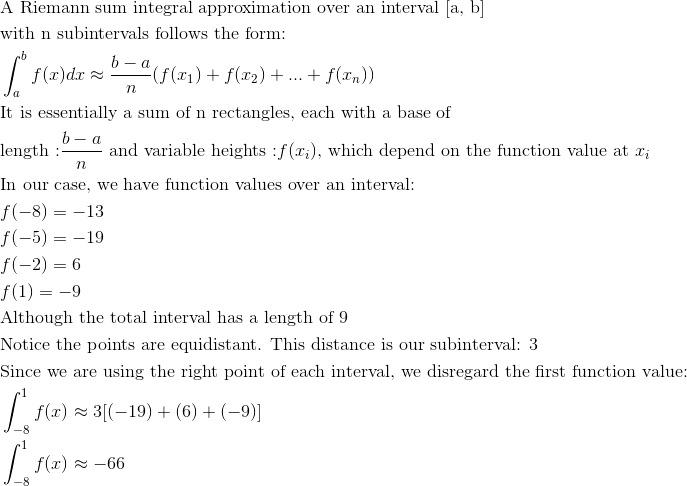Example Question #3 : Riemann Sums (Left, Right, And Midpoint Evaluation Points)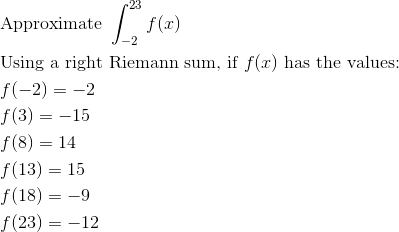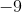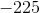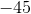Explanation: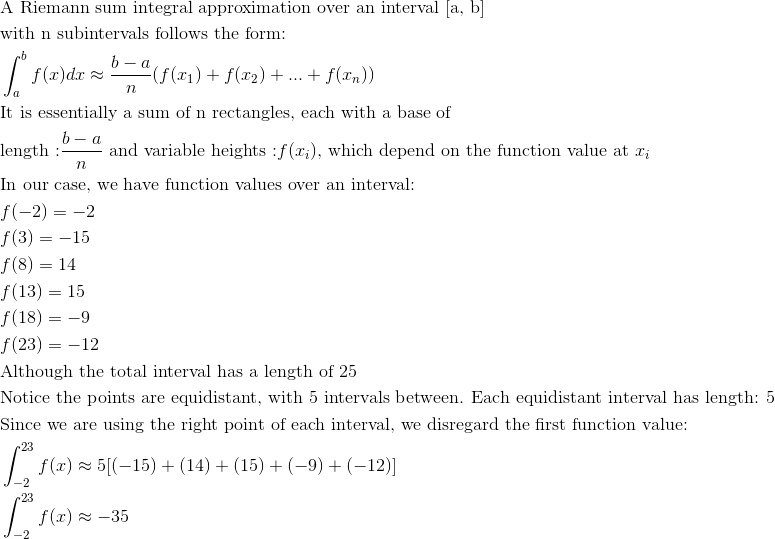Example Question #4 : Riemann Sums (Left, Right, And Midpoint Evaluation Points)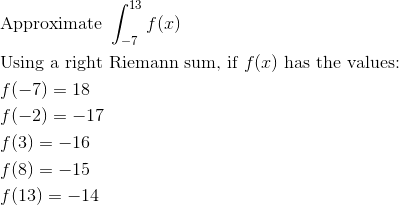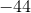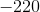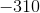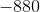Explanation: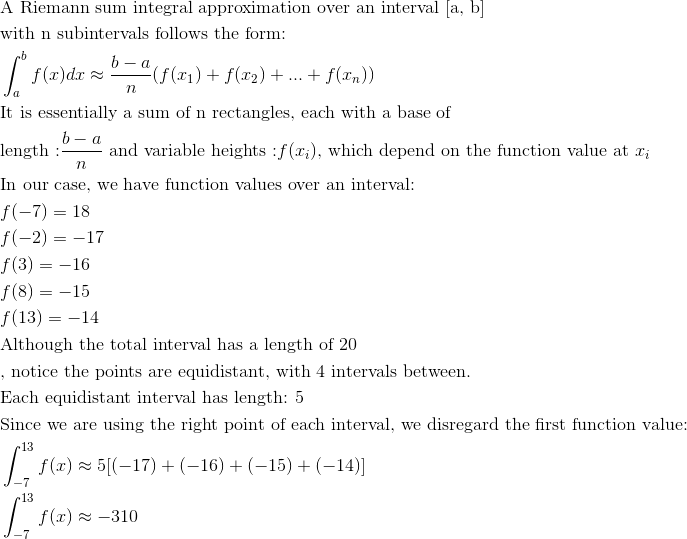Example Question #5 : Riemann Sums (Left, Right, And Midpoint Evaluation Points)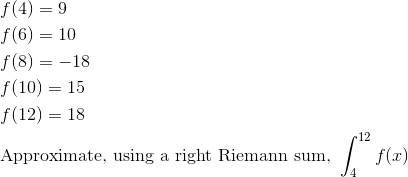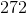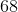Explanation: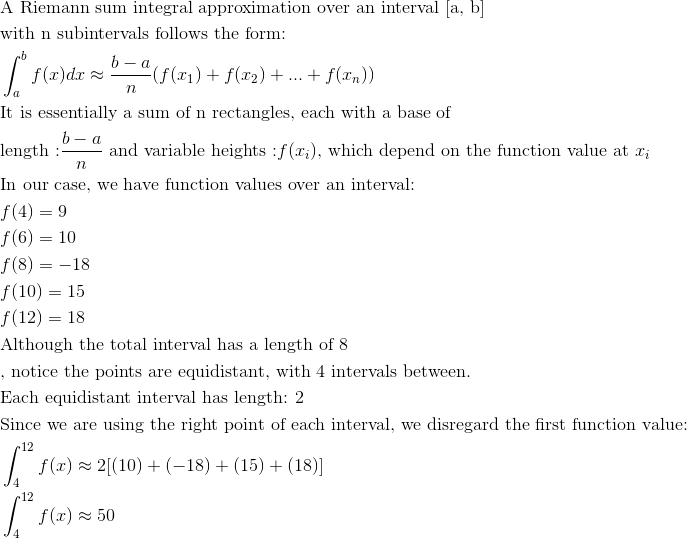Example Question #6 : Riemann Sums (Left, Right, And Midpoint Evaluation Points)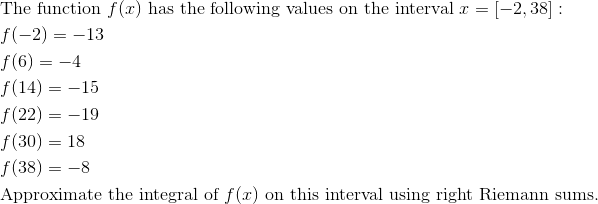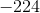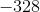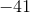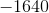Explanation: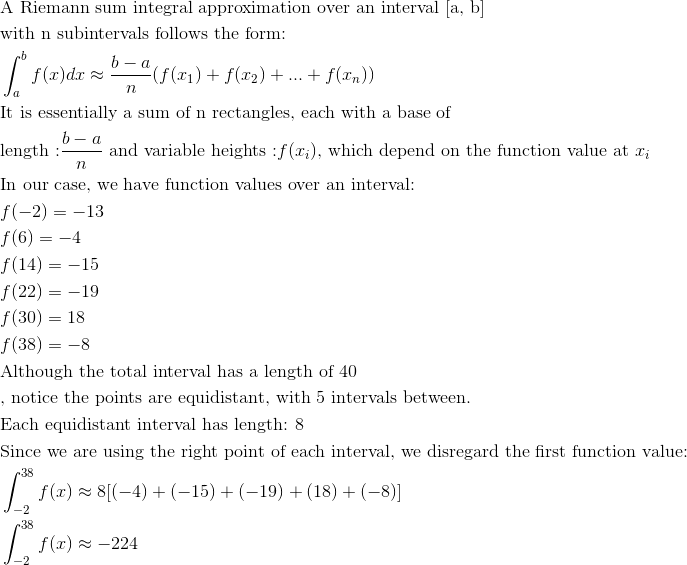Example Question #7 : Riemann Sums (Left, Right, And Midpoint Evaluation Points)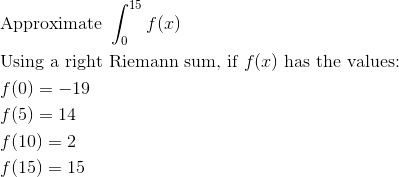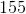Explanation: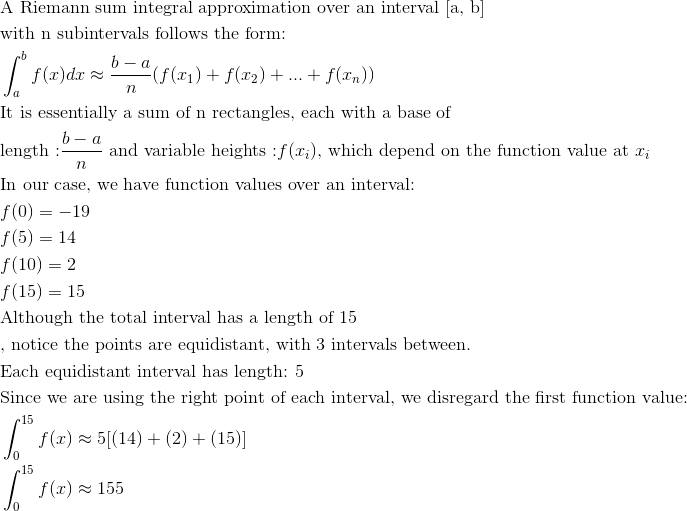Example Question #8 : Riemann Sums (Left, Right, And Midpoint Evaluation Points)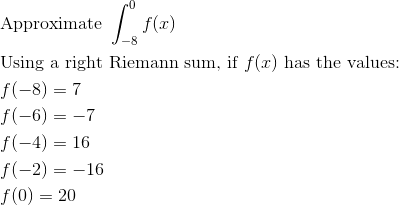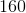Explanation: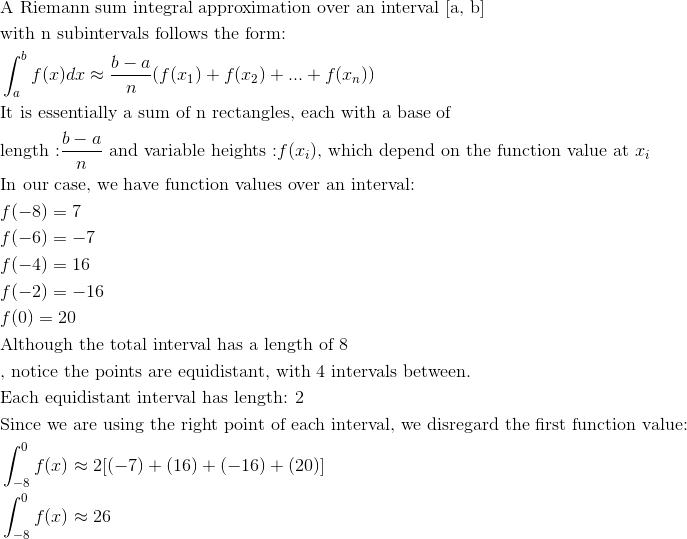Example Question #9 : Riemann Sums (Left, Right, And Midpoint Evaluation Points)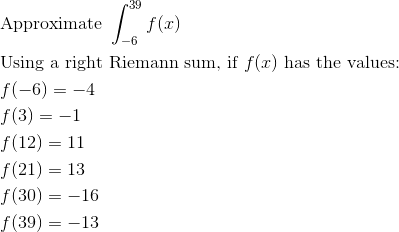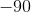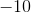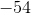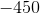Explanation: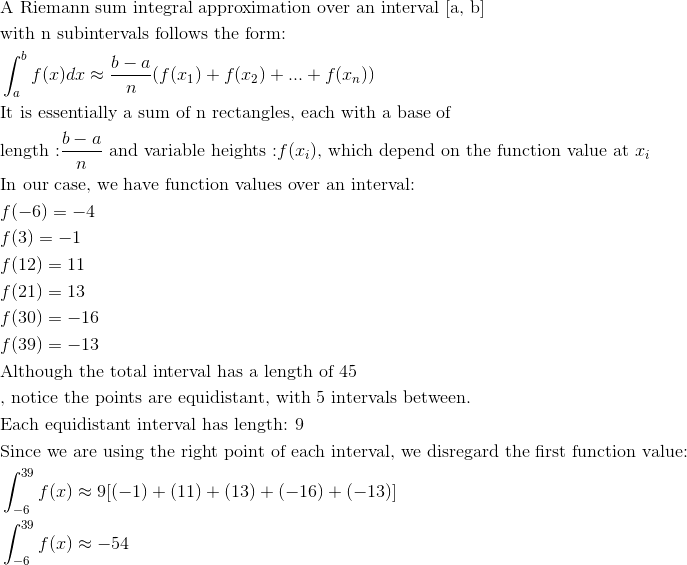Example Question #10 : Riemann Sums (Left, Right, And Midpoint Evaluation Points)